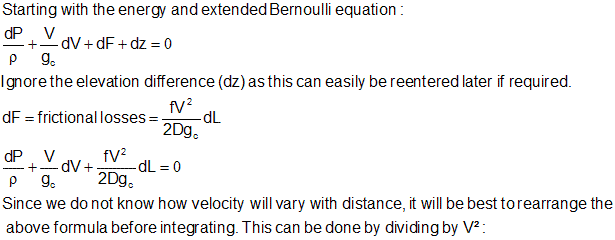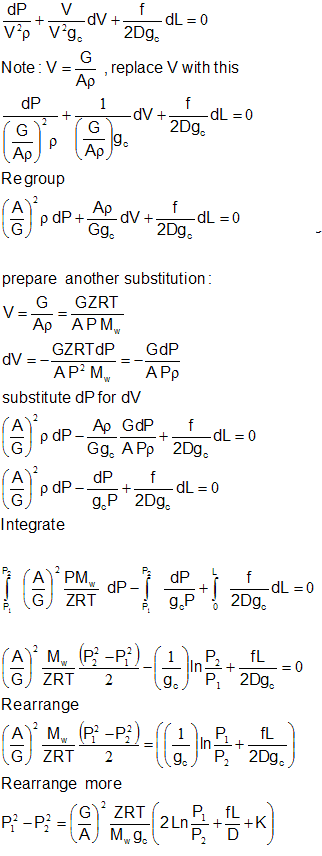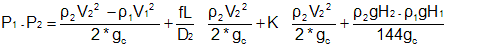Oil and Gas ProcessingFluid Flow
Isothermal Compressible Flow

For cases which have a constant pipe cross sectional area the following is a derivation a formula for calculating large pressure drops for isothermal compressible flow:For cases which have changes in pipe cross sectional areas, the method must be simplified. In these case the problem should be divided into several segments. The change in pipe size should be modeled by itself, with little or no pipe length (just the change in pipe size and the loss incurred in the reducer or expander).

This is simply the Bernoulli equation:

DP total= DP velocity head+ DP friction+ DP fitting+ DP elevationNote in the equation above the pipe length and fitting losses are based on the downstream (2) diameter.

 A = Area, ft² D = Pipe inside diameter, ft F = Friction factor (Darcy) G = Mass Flow rate, lb/sec g c = 32.174 ft-lb m/sec²-lb f K = Fitting K factor L = Pipe length, ft ln = Natural logarithm M w = Gas molecular weight (lbs) P = Pressure, lb/ft² R = Gas constant, (lbf/ft²)(ft³/mole-°R) T = Temperature, °Rankine V = Vel, ft/sec Z = Inlet Compressibility

Subscript 1 and 2 refer to inlet and outlet conditions respectively

Fitting K factor

The fitting K factor is an empirically derived factor to account of pressure losses encountered as a result of pipe fittings, such as valves, elbows, tees, reducers and enlargers. The K factor for the same type of fitting is similar for different pipe sizes. Algorithms have been developed though to account for the small changes. Also the CV factor use to characterize valves can be converted to a K value to allow for the summing of all of the fittings in a pipe.

Values for K can be easily found in Perry’s Chemical Engineers handbook, Crane, etc.

Special situations where the flow divides in a tee are not commonly documented. As an example for flow going from a 20 inch line into a 10 inch side branch, the K value could be calculated as a “hard” tee ( approximately 1) and a sudden reduction. This would be correct if all of the flow goes from the 20 inch line to the 10 inch line. In most cases this does not occur. In a manifold situation only some of the flow will go to the 10 inch line. In that case the calculated K for the reducer should be based on the change in velocity from the 20 inch line to the 10 inch line. If the velocity upstream of the tee is the same as the velocity in the 10 inch line there should be no additional K value added to the calculation (in addition to the K value for the hard tee).

The following formula can be used to calculate the pressure drop for flowing from a line to the branch on a tee:

D 2 = D 1 (V1/V 2) ½

The diameter calculated from the above algorithm can then be used to calculate the beta ( b ) in the abrupt reducer calculation:

K 2 = 0.5 * (1 – b2)/ b 4

The K 2 in the above calculation is the K for the smaller pipe (the branch tee). If the velocity is lower in the branch than in the run, then the calculation will be an expansion:

K 2 = (1 – b2)/ b 4

Generalized table of K values

 45° Elbow (std, r/d=1) 0.22 45° Elbow (std, r/d=1) 0.16 90° Elbow (std, r/d=1) 0.43 90° Elbow (long radius, r/d=1.5) 0.27 Tee used as elbow 1 Tee, run 0.54 Gate Valve 0.11 Ball Valve (reduced port) 0.16 Globe 4.34 Butterfly 0.27 Lift Check Valve 10.8 Swing Check Valve 1.63 Sharp Entrance 0.5 Pipe Exit 1

For detailed calculations you should get the actual cv’s for the valves and either calculate the pressure drop directly or convert to a K value and sum with the other fittings.

Other fitting type pressure drops not listed above are those associated with flow meters, restriction orifices, perforated plates, etc.

It should also be noted that the above K values are for fluid flow in the turbulent flow. Laminar flow can have K values that are up to 100 times higher. The typical case for laminar flow in the oil and gas production business is when you are dealing with viscous crude oils, or fuel oils. Fuel oil number 6 ( bunker C) is a good example of a very viscous fluid, that will be in the laminar flow region for most applications (unless you heat it up to over 200°F, which is necessary for most operations).

Friction Factor

The friction factor ( Darcy or Moody ) can be read off of a chart or calculated. It should be noted that all of the friction factors used here are the Darcy or Moody, not the Fanning friction factor. The Fanning friction factor is ¼ of the value of the Darcy friction factor. The pressure drop formula is the same, with the exception that it has an additional coefficient (4). The results calculated will be the same, it is just important to make sure you do not mix the Darcy friction factor with an equation set up for the Fanning friction factor.

Roughness Factor

This is a measure of the smoothness of the pipewall. It is necessary to calculated the friction factor. Below is a list of the typical ones:

Cast Iron = 0.00085 ft

Galvanized Iron= 0.0005 ft

Asphalted Cast Iron = 0.0004 ft

Commercial Steel or Wrought Iron = 0.00015 ft

Drawn Tubing = 0.000005 ft

Fiber Glass = 0.00015 to 0.000017 ft

Roughness factor is sometimes provided in inches, and may require conversion to feet depending on the graph or formula you are using.

body2